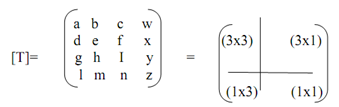## 3-d transformation, Computer Graphics

Assignment Help:

3-D Transformation

The capability to represent or display a three-dimensional object is basically to the knowing of the shape of that object. Moreover, the capability to rotate, translate and also project views of such object is also, in various cases, basically to the understanding of its shape. Manipulation, construction and viewing of 3-dimensional graphic images need the utilization of coordinate transformations and 3-dimensional geometric. Within geometric transformation, the coordinate system is set and the desired transformation of the object is finished w.r.t. the coordinate system. During coordinate transformation, the object is fixed and the preferred transformation of the object is complete on the coordinate system itself. Such transformations are formed via composing the essential transformations of translation, rotation and scaling. All of these transformations can be demonstrated as a matrix transformation. It permits more complex transformations to be constructed by utilization of matrix concatenation or multiplication. We can make the complicated objects/pictures, via immediate transformations. In order to demonstrate all these transformations, we require utilizing homogeneous coordinates.

Thus, if P(x,y,z) be any point in 3-dimensional space then in Homogeneous coordinate system, we add a fourth-coordinate to a point. It is in place of (x,y,z), all points can be represented via a Quadruple (x,y,z,H), where H≠0; along with the condition is x1/H1=x2/H2; y1/H1=y2/H2; z1/H1=z2/H2. For two points (x1, y1, z1, H1) = (x2, y2, z2, H2) ; such that H1 ≠ 0, H2 ≠ 0. Hence any point (x,y,z) in Cartesian system can be illustrated by a four-dimensional vector like (x,y,z,1) in HCS. Similarly, if (x,y,z,H) be any point in Homogeneous coordinate system then (x/H,y/H,z/H) be the equivalent point in Cartesian system. Hence, a point in 3-dimensional space (x,y,z) can be demonstrated by a four-dimensional point as: (x',y',z',1)=(x,y,z,1).[T], here [T] is several transformation matrix and (x',y'z',1) is a new coordinate of a specified point (x,y,z,1), so after the transformation.

The completed 4x4 transformation matrix for 3-dimensional homogeneous coordinates as:The upper left (3x3) sub matrix generates scaling, reflection, rotation and shearing transformation. The lower left (1x3) sub-matrix generates translation and the upper right (3x1) sub-matrix produces a perspective transformation that we will study in the subsequent section. The final lower right-hand (1x1) sub-matrix generates overall scaling.

#### Image - based rendering, Explore and understand light field. Checking out o...

Explore and understand light field. Checking out one of the image libraries. 1. You can modify existing source code, or develop your own to achieve light field rendering; 2.

#### Important points for designing the animation sequence, Important Points for...

Important Points for Designing the Animation Sequence There are several applications which do not follow this sequence as, real time computer animations generated by vehicle dr

#### Advantages of computer aided design, Advantages of Computer aided design  ...

Advantages of Computer aided design  -   It is simpler to modify drawings  -   A library of parts can be kept  -   Ability to do automatic costings -   Ability to mod

#### What is scaling and shearing, What is scaling and shearing? The scaling...

What is scaling and shearing? The scaling transformations alters the shape of an object and can be carried out  by multiplying every vertex (x,y) by scaling factor Sx, Sy where

#### Removing polygons hidden through a surrounding polygon, Removing Polygons H...

Removing Polygons Hidden through a Surrounding Polygon: The key to capable visibility calculation lies actually a polygon is not visible whether it is in back of a surrounding

#### Z-buffer algorithm, How to implement z-buffer algorithm using C programming...

How to implement z-buffer algorithm using C programming

#### What do you understand by complementary colors, Problem : a. (i) Giv...

Problem : a. (i) Give another name for adjacent color. (ii) Describe briefly what do you understand by an adjacent color? b. (i) Describe briefly what do you unders

#### Z-buffer, describe z-buffer algorithm removing hidden surface

describe z-buffer algorithm removing hidden surface

vecgen algorithm

#### Algorithm scan-line, Algorithm (scan-line): For each scan line perform ...

Algorithm (scan-line): For each scan line perform from step (1) to (3). 1) For all pixels on a scan-line, arrange depth [x]=1.0 i.e. max value and Intensity [x] = background